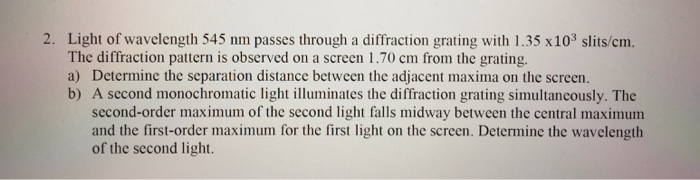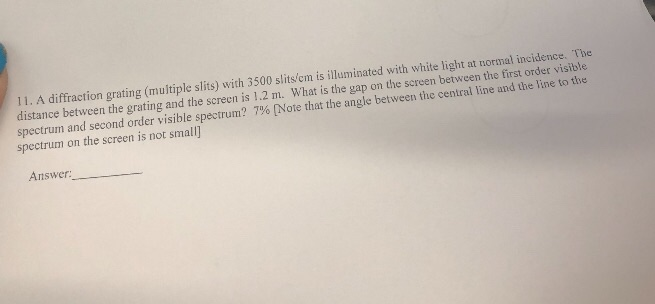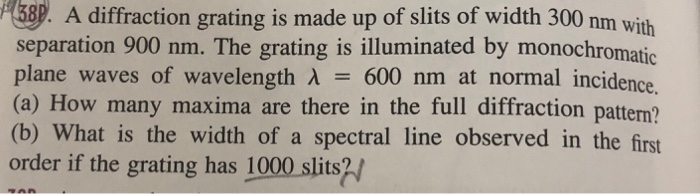Question

A 4.4-cm-wide diffraction grating has 2400 slits. It is illuminated by light of wavelength 530 nm .

What is the angle (in degrees) of the first diffraction order?

What is the angle (in degrees) of the second diffraction order?

What is the angle (in degrees) of the first diffraction order

angle = wavelength divided by grating = .00000053/ (.044/ 2400 ) = 0.028 radians = 1.60 degrees

What is the angle (in degrees) of the second diffraction order

the second one is just twice that 0.056 radians = 3.20 degrees

#### Earn Coins

Coins can be redeemed for fabulous gifts.

Similar Homework Help Questions
• ### A 1.6-cm-wide diffraction grating has 1000 slits. It is illuminated by light of wavelength 650 nm....

A 1.6-cm-wide diffraction grating has 1000 slits. It is illuminated by light of wavelength 650 nm. What are the angles of the first two diffraction orders?

• ### A diffraction grating is 1.50 cm wide and contains 2400 lines

A diffraction grating is 1.50 cm wide and contains 2400 lines. When used with light of a certain wavelength, a third-order maximum is formed at an angle of 14.5°. What is the wavelength in nm?

• ### 2. Light of wavelength 545 nm passes through a diffraction grating with 1.35 x103 slits/cm The...2. Light of wavelength 545 nm passes through a diffraction grating with 1.35 x103 slits/cm The diffraction pattern is observed on a screen 1.70 cm from the grating. a) Determine the separation distance between the adjacent maxima on the screen. b) A second monochromatic light illuminates the diffraction grating simultancously. The second-order maximum of the second light falls midway between the central maximum and the first-order maximum for the first light on the screen. Determine the wavelength of the second...

• ### A 500 lines per mm diffraction grating is illuminated by light of wavelength 560 nm ....

A 500 lines per mm diffraction grating is illuminated by light of wavelength 560 nm . Part A What is the maximum diffraction order seen Part B: What is the angle of each diffraction order starting from zero diffraction order to the maximum visible diffraction order? Enter your answers in degrees in ascending order separated by commas.

• ### A grating with 320 slits/centimeter is illuminated with light of wavelength 493 nm. (a) What is...

A grating with 320 slits/centimeter is illuminated with light of wavelength 493 nm. (a) What is the highest order number that canbe observed with this grating? (b) Repeat for a grating of 1376 slits/centimeter.

• ### A diffraction grating with 750 slits per mm is illuminated by light which gives a first-order...

A diffraction grating with 750 slits per mm is illuminated by light which gives a first-order diffraction angle of 34.0°. For the steps and strategies involved in solving a similar problem, you may view a Video Tutor Solution.    Part A What is the wavelength of the light?

• ### 11. A diffraction grating (multiple slits) with 3500 slits/em is illuminated with white light at normal...11. A diffraction grating (multiple slits) with 3500 slits/em is illuminated with white light at normal incidence. The distance between the grating and the screen is 1.2 m. What is the gap on the screen between the first order visible spectrum and second order visible spectrum? 7% [Note that the angle between the central line and the line to the spectrum on the screen is not small] Answer:

• ### A diffraction grating is made up of slits of width 300 nm with separation 900 nm....A diffraction grating is made up of slits of width 300 nm with separation 900 nm. The grating is illuminated by monochromatic plane waves of wavelength λ 600 nm at normal incidence (a) How many maxima are there in the full diffraction pattern? (b) What is the width of a spectral line observed in the first order if the grating has 1000 slits?/

• ### A 500 lines per mm diffraction grating is illuminated by light of wavelength 510...continues

A 500 lines per mm diffraction grating is illuminated by light of wavelength 510 nm. What is the angle of each diffraction order?

• ### A 500 lines per mm diffraction grating is illuminated by light of wavelength 640 nm .

A 500 lines per mm diffraction grating is illuminated by light of wavelength 640 nm . For the steps and strategies involved in solving a similar problem, you may view a Video Tutor Solution.Part AWhat is the maximum diffraction order seen? Express your answer as an integer. Part B What is the angle of each diffraction order starting from zero diffraction order to the maximum visible diffraction order?